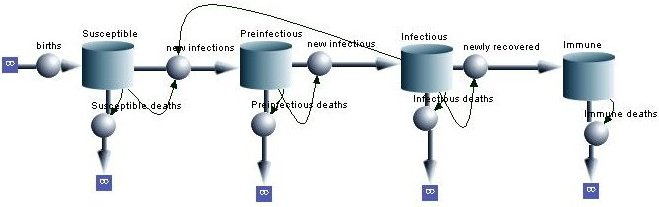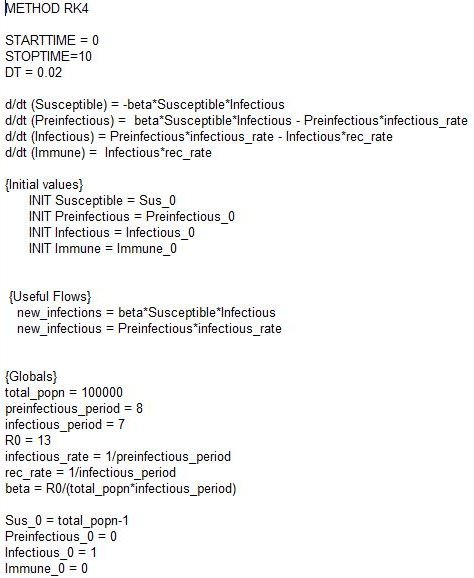# Chapter 3

## How are models set up? An introduction to differential equations

This chapter provides an introduction to the methods for writing down differential equations. In contrast with difference equations, which describe events occurring at discrete time intervals, differential equations describe events occurring in continuous time.

The files accompanying this chapter provide an introduction to the user-friendly package Berkeley Madonna, which allows users to set up models either by drawing the model using a flowchart or using an equation editor.An illustration of a model set up in Berkeley Madonna using the flowchart and the equation editor.

The flowchart option allows users to set up models quickly, using few equations. This is particularly helpful for readers who prefer to visualize their model or those who are uncomfortable with using equations.

Readers who prefer to work with equations can use the equation editor to create models.